# Esa Helttula 提供アプリ一覧

[ 教育 ]
This math app is about the concept of order of operations. The user can evaluate random and custom expressions with exponentiation, division, multiplication,...
[ 教育 ]
Wired.com: "It’s fantastic for helping kids (and grown-ups) practice polynomial manipulation and division. I can’t recommend Esa’s math apps enough." With t...
[ 教育 ]
With the Polynomial Addition and Subtraction app you can practice adding and subtracting polynomials with a step-by-step method. And you can get additional h...
[ 教育 ]
With the Polynomial Multiplication app you can practice multiplying polynomials with a step-by-step method. And you can get additional help at each step. Th...
[ 教育 ]
The Fraction as Slope app provides algebra students a new visual way to think about slope and fractions by showing how fractions are slopes. This provides an...
[ 教育 ]
The Long Division app has been featured by Apple in "New & Noteworthy", "Math - Numbers & Operations", and in "Math Apps for Middle School". Educational App ...
[ 教育 ]
This math app is about visually exploring how percentages and decimals are just special kinds of fractions with their own notation. There are no tasks to com...
[ 教育 ]
This math app can be used to teach and study the Russian multiplication method. The user can solve random or custom multiplication problems. The number of di...
[ 教育 ]
This math app can be used to teach and study the column division method. The app is easy to use and it has an intuitive interactive interface with customizab...
[ 教育 ]
This math app can be used to teach and study the grid multiplication method. The app is easy to use and it has an intuitive interactive interface with custom...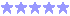1人
[ 教育 ]
This app can be used to teach and study the alternative column-addition method used in the Everyday Mathematics curriculum developed by the University of Chi...
[ 教育 ]
This math app can be used to teach and study the rounding of whole numbers. You can set your own problems or solve random problems. The numbers to be rounded...
[ 教育 ]
In the Opposite-Change Addition app the user can keep adding a number to one addend and subtract the same number from the other addend until the addition pro...
[ 教育 ]
In the Same-Change Subtraction app the user can keep adding or subtracting the same amount from both numbers until the subtraction problem can be solved easi...
[ 教育 ]
The counting-up subtraction app lets students solve subtraction problems by counting up from the lesser number step by step. The first step is to get to the ...
[ 教育 ]
Explore and learn the multiplication table in a visual way. Wired.com: "I highly recommend it for any kid who is learning their multiplication tables". Fou...
[ 教育 ]
This math app can be used to teach and study the column subtraction method. The app is easy to use and it has an intuitive interactive interface with customi...
[ 教育 ]
The Fraction Math app can be used to teach and study fractions by solving addition, subtraction, division and multiplication problems with fractions step by ...
[ 教育 ]
This math app can be used to teach and study the equal addition subtraction method. The user can solve random or custom subtraction problems with small and l...
[ 教育 ]
This math app is about a multiplication technique introduced by Shri Bharti Krishna Tirthaji in his book “Vedic Mathematics”, published in 1965. Tirthaji cla...
[ 教育 ]
This universal app is part of a set of idevbooks math apps. Reviewed by Wired.com: "A Set of Excellent iPhone Math Apps for the Older Child”. The Long Mult...
[ 教育 ]
This app can be used to teach and study the ancient lattice multiplication method. Solving lattice multiplication problems is also excellent times tables pra...
[ 教育 ]
This math app can be used to teach and study the partial quotients division method. The app is easy to use and it has an intuitive interactive interface with...
[ 教育 ]
This app can be used to teach and study the ancient Egyptian multiplication method. The Egyptian Multiplication Method Ancient Egyptians were able to mult...
[ 教育 ]
This math app can be used to teach and study the partial products multiplication method. The app is easy to use and it has an intuitive interactive interface...
[ 教育 ]
This math app can be used to teach and study the column addition method. The app is easy to use and it has an intuitive interactive interface with customizab...
[ 教育 ]
Understanding the concept of places and values in the base then system is essential for long-term understanding. In addition place value is essential to the ...
[ 教育 ]
Understanding the concept of places and values in the base then system is essential for long-term understanding. In multiplication place value is essential t...
[ 教育 ]
This math app can be used to teach and study the partial quotients division method. The app is easy to use and it has an intuitive interactive interface with...
[ 教育 ]
This math app can be used to teach and study the partial products multiplication method. The app is easy to use and it has an intuitive interactive interface...
[ 教育 ]
This math app can be used to teach and study the column addition method. The app is easy to use and it has an intuitive interactive interface with customizab...
[ 教育 ]
This app can be used to teach and study the partial sums addition method. The app is easy to use and it has an intuitive interactive interface with customiza...
[ 教育 ]
This math app can be used to teach and study the rounding of decimals. You can set your own problems or solve random problems. The decimal numbers can be rou...
[ 教育 ]
The Swedish Long Division app can be used to teach and study the Swedish long division method. The user can solve custom and random division problems with sm...
[ 教育 ]
This app can be used to teach and study the partial sums addition method. The app is easy to use and it has an intuitive interactive interface with customiza...
[ 教育 ]
The German Long Division app can be used to teach and study the German long division method. The user can solve custom and random division problems with smal...
[ 教育 ]
This math app can be used to teach and study the rounding of whole numbers. You can set your own problems or solve random problems. The numbers to be rounded...
[ 教育 ]
This math app can be used to teach and study the column subtraction method. The app is easy to use and it has an intuitive interactive interface with customi...
[ 教育 ]
This math app is about a multiplication technique introduced by Shri Bharti Krishna Tirthaji in his book “Vedic Mathematics”, published in 1965. Tirthaji cla...
[ 教育 ]
This math app can be used to teach and study the rounding of decimals. You can set your own problems or solve random problems. The decimal numbers can be rou...
[ 教育 ]
This math app can be used to teach and study the partial differences subtraction method. The app is easy to use and it has an intuitive interactive interface...
[ 教育 ]
This math app can be used to teach and study the column division method. The app is easy to use and it has an intuitive interactive interface with customizab...
[ 教育 ]
The European Long Division app can be used to teach and study the long division method that is used in several European countries. The user can solve custom ...
[ 教育 ]
This math app can be used to teach and study the grid multiplication method. The app is easy to use and it has an intuitive interactive interface with custom...
[ 教育 ]
The Long Division app can be used to teach and study the long division method. Solving long division problems is also excellent subtraction and times tables ...
[ 教育 ]
Understanding the concept of places and values in the base then system is essential for long-term understanding. Division using place value discs emphasizes ...
[ 教育 ]
This app can be used to teach and study the trade-first subtraction method. The app is easy to use and it has an intuitive interactive interface with customi...
[ 教育 ]
This math app can be used to teach and study the partial differences subtraction method. The app is easy to use and it has an intuitive interactive interface...
[ 教育 ]
This app can be used to teach and study the trade-first subtraction method. The app is easy to use and it has an intuitive interactive interface with customi...
[ 教育 ]
This math app can be used to teach and study the left-to-right subtraction method. The app is easy to use and it has an intuitive interactive interface with ...
[ 教育 ]
This app can be used to teach and study the ancient lattice multiplication method. Solving lattice multiplication problems is also excellent times tables pra...
[ 教育 ]
This app is part of a set of idevbooks math apps for iOS and Mac. Reviewed by Wired.com: "A Set of Excellent iPhone Math Apps for the Older Child”. The Lon...
[ 教育 ]
The Dutch Long Division app can be used to teach and study the Dutch long division method. The user can solve custom and random division problems with small ...
[ 教育 ]
Explore division of one unit into fractions with multi-touch. Touch any fraction of the unit with two or more fingers to further divide it by the number of t...
[ 教育 ]
Understanding the concept of places and values in the base then system is essential for long-term understanding. In subtraction place value is essential to t...オススメ！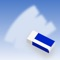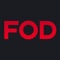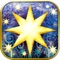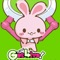お得情報ピックアップ！49%値引中41%値引中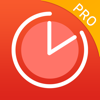68%値引中51%値引中67%値引中Now loading...